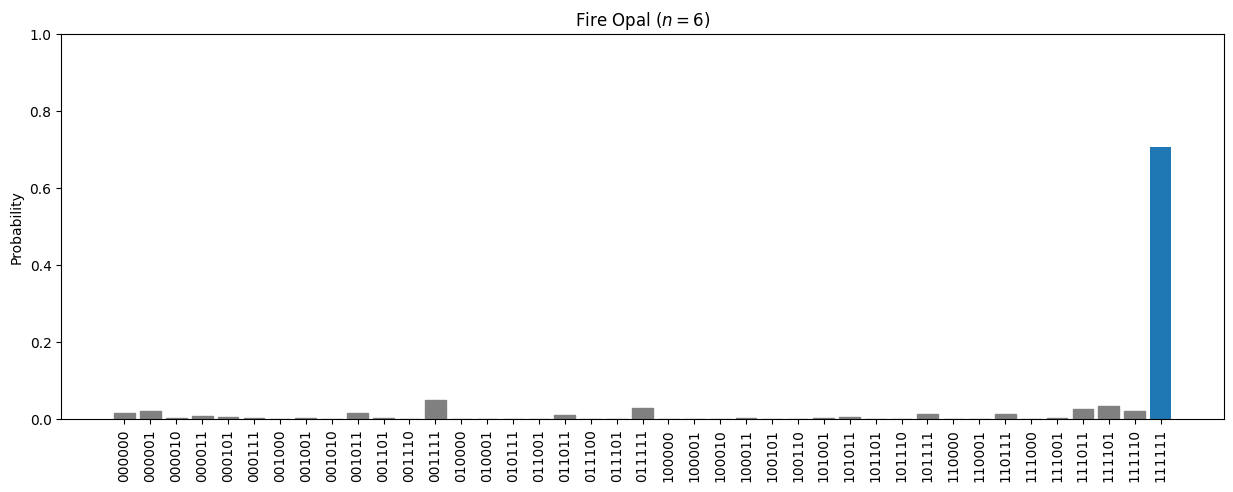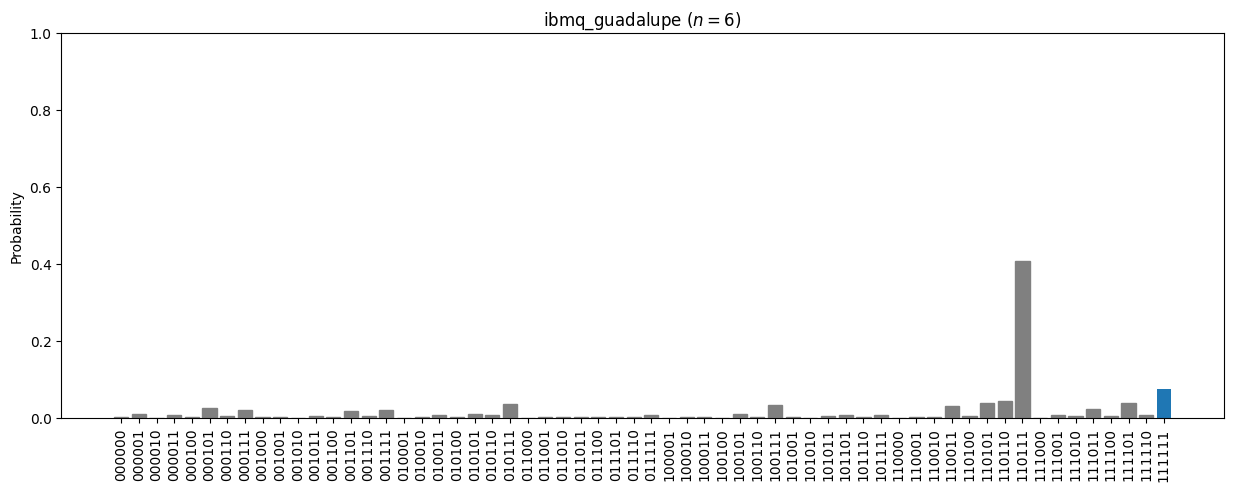•Fire Opal
• Get started

# Get started

Fire Opal at a glance and quickstart

## Fire Opal overview

Fire Opal is a Python package that applies a complete suite of error suppression techniques to vastly improve the quality of quantum algorithm results, often transforming quantum computer outputs from random to useful. For an in-depth explanation of Fire Opal's benefits and capabilities, check out the Fire Opal overview.

This tutorial will run through the steps to set up Fire Opal and use it to run a Bernstein–Vazirani circuit. After completion, you will have demonstrated Fire Opal's benefits by comparing the success probabilities of executing the circuit with both Fire Opal and Qiskit.

## Setup

You will need to sign up for a Q-CTRL account to run the Fire Opal package.

### 2. Install Fire Opal Python package

Refer to how to set up your environment and install Fire Opal for more detailed information on how to set up your development environment to run Fire Opal. If you already have Python and a package manager installed, you can use the following command to install the necessary packages:

pip install fire-opal qiskit matplotlib qiskit-ibm-provider
import fireopal
import qiskit
from qiskit_ibm_provider import IBMProvider
import matplotlib.pyplot as plt
import os

If you are a member of multiple organizations, you must specify which organization to use by setting the organization parameter, as shown below.

fireopal.config.configure_organization(organization_slug="organization_slug")

where organization_slug is the unique ID used to identify this organization. You can check organization names and other details by visiting your Q-CTRL account.

While Fire Opal's techology is inherently backend agnostic, in this tutorial we will run the circuit on an IBM Quantum backend device.

Note: IBM Quantum offers public access to some of their quantum computers. However, queue times for public systems can be long, which will cause delays in the execution steps of the demo (Step 5 and Step 7).

These delays are extraneous to Fire Opal, and they can be avoided only by reserving system time, which can be done by joining the IBM Quantum Network. This will also provide access to premium IBM systems that are less highly utilized.

## Demo: Running the Bernstein–Vazirani algorithm with Fire Opal

We'll use Fire Opal to run a Bernstein–Vazirani circuit. This algorithm is broadly used to find a string from the outputs of a black box function, though this information is not necessary for the sake of running this example. In this demo, we will simply demonstrate how Fire Opal can increase the likelihood of algorithmic success, by a degree of more than 25-fold for this algorithm.

### 1. Define helper functions

We will start by defining two helper functions:

• draw_circuit: draws our QASM circuit
• plot_bv_results: plots the results of our experiments
shot_count = 2048

def draw_circuit(qasm_str: str):
"""Draws a QASM circuit."""
circuit = qiskit.QuantumCircuit.from_qasm_str(qasm_str)
print(circuit)

def plot_bv_results(results, hidden_string, title=""):
"""Plot a probability histogram and highlight the hidden string."""
bitstrings = sorted(results.keys())

def to_probability(value, total):
if isinstance(value, float):
return value
return value / total

probabilities = [to_probability(results[b], shot_count) for b in bitstrings]
plt.figure(figsize=(15, 5))
bars = plt.bar(bitstrings, probabilities)
plt.xticks(rotation=90)

for index, bitstring in enumerate(bitstrings):
if bitstring != hidden_string:
bars[index].set_color("grey")

plt.ylabel("Probability")
plt.ylim([0, 1])
plt.title(title)
plt.show()

### 2. Provide the quantum circuit

Here, we will define the Bernstein–Vazirani circuit as an OpenQASM string and visualize it using our previously defined helper function draw_circuit. Such a string can also be generated by exporting a quantum circuit written with any quantum-specific Python library.

circuit_qasm = """OPENQASM 2.0;
include "qelib1.inc";

// Define the 7-qubit BV oracle 'f'. For each '1' in the hidden string,
// at bit location 'i', we act with the CX between qubit 'i' and the
// last qubit.
gate f q0, q1, q2, q3, q4, q5, q6 { // hidden string '111111'
cx q0, q6;
cx q1, q6;
cx q2, q6;
cx q3, q6;
cx q4, q6;
cx q5, q6;
}

// Define the quantum register and the classical register
qreg qubit;
creg cbits;

// Setup rest of the circuit
h qubit;
z qubit;
f qubit, qubit, qubit, qubit, qubit, qubit, qubit;
h qubit;

// Measure the hidden string.
measure qubit -> cbits;
measure qubit -> cbits;
measure qubit -> cbits;
measure qubit -> cbits;
measure qubit -> cbits;
measure qubit -> cbits;
"""
draw_circuit(circuit_qasm)
         ┌───┐     ┌────┐┌───┐┌─┐
qubit_0: ┤ H ├─────┤0   ├┤ H ├┤M├───────────────
├───┤     │    │├───┤└╥┘┌─┐
qubit_1: ┤ H ├─────┤1   ├┤ H ├─╫─┤M├────────────
├───┤     │    │├───┤ ║ └╥┘┌─┐
qubit_2: ┤ H ├─────┤2   ├┤ H ├─╫──╫─┤M├─────────
├───┤     │    │├───┤ ║  ║ └╥┘┌─┐
qubit_3: ┤ H ├─────┤3 f ├┤ H ├─╫──╫──╫─┤M├──────
├───┤     │    │├───┤ ║  ║  ║ └╥┘┌─┐
qubit_4: ┤ H ├─────┤4   ├┤ H ├─╫──╫──╫──╫─┤M├───
├───┤     │    │├───┤ ║  ║  ║  ║ └╥┘┌─┐
qubit_5: ┤ H ├─────┤5   ├┤ H ├─╫──╫──╫──╫──╫─┤M├
├───┤┌───┐│    │├───┤ ║  ║  ║  ║  ║ └╥┘
qubit_6: ┤ H ├┤ Z ├┤6   ├┤ H ├─╫──╫──╫──╫──╫──╫─
└───┘└───┘└────┘└───┘ ║  ║  ║  ║  ║  ║
cbits: 6/══════════════════════╩══╩══╩══╩══╩══╩═
0  1  2  3  4  5


### 3. Provide your device information and credentials

Next, we'll provide device information for the real hardware backend. Fire Opal will execute the circuit on the backend on your behalf, and it is designed to work seamlessly across multiple backend providers. For this example, we will use an IBM Quantum hardware device.

Note that the code below requires your IBM Quantum API token. Visit IBM Quantum to sign up for an account and learn how to access systems with your account.

Use the obtained credentials to replace "YOUR_IBM_TOKEN".

# These are the properties for the publicly available provider for IBM backends.
# If you have access to a private provider and wish to use it, replace these values.
hub = "ibm-q"
group = "open"
project = "main"
token = "YOUR_IBM_TOKEN"
credentials = fireopal.credentials.make_credentials_for_ibmq(
token=token, hub=hub, group=group, project=project
)

IBMProvider.save_account(
token, overwrite=True, instance=hub + "/" + group + "/" + project
)
provider = IBMProvider()

Next we will use the function show_supported_devices to list the devices that are both supported by Fire Opal and accessible to you when using the credentials above.

supported_devices = fireopal.show_supported_devices(credentials=credentials)[
"supported_devices"
]
for name in supported_devices:
print(name)
ibm_lagos
ibmq_belem
ibmq_quito
ibmq_manila
ibmq_lima
ibm_nairobi
ibmq_jakarta
ibm_perth


From the resulting list, you can choose a backend device and replace "desired_backend". This list will not include any devices outside of those accessible to you. You can check your accessible devices through your IBM Quantum account.

# Enter your desired IBM backend here or select one with a small queue
backend_name = "desired_backend"
print(f"Will run on backend: {backend_name}")

### 4. Validate the circuit and backend

Now that we have defined our credentials and are able to select a device we wish to use, we can validate that Fire Opal can compile our circuit, and that it's compatible with the indicated backend.

validate_results = fireopal.validate(
circuits=[circuit_qasm], credentials=credentials, backend_name=backend_name
)

if validate_results["results"] == []:
print("No errors found.")
else:
print("The following errors were found:")
for error in validate_results["results"]:
print(error)
No errors found.


In this previous example, the output should be an empty list since there are no errors in the circuit, i.e. validate_results["results"] == []. Note that the length of the validate_results list is the total number of errors present across all circuits in a batch. Since our circuit is error free, we can execute our circuit on real hardware.

### 5. Execute the circuit using Fire Opal

In the absence of hardware noise, only a single experiment would be required to obtain the correct hidden string: 111 111. However in real quantum hardware, noise disturbs the state of the system and degrades performance, decreasing the probability of obtaining the correct answer for any single experiment. Fire Opal automates the adjustments made by experts when running circuits on a real device.

print(
"Submitted the circuit to IBM. Note: there may be a delay in getting results due to IBM "
"device queues. Visit https://quantum-computing.ibm.com/jobs to check your queue position."
)
real_hardware_results = fireopal.execute(
circuits=[circuit_qasm],
shot_count=shot_count,
credentials=credentials,
backend_name=backend_name,
)

bitstring_results = real_hardware_results["results"]
Submitted the circuit to IBM. Note: there may be a delay in getting results due to IBM device queues. Visit https://quantum-computing.ibm.com/jobs to check your queue position.


### 6. Analyze results

Now you can look at the outputs from the quantum circuit executions. The success probability is simply the number of times the hidden string was obtained out of the total number of circuit shots. For reference, running this circuit on a real device without Fire Opal typically has a success probability of 2-3%. As you can see, Fire Opal greatly improved the success probability.

print(f"Success probability: {100 * bitstring_results['111111']}")
plot_bv_results(
bitstring_results, hidden_string="111111", title=f"Fire Opal ($n=6$)"
)
Success probability: 70.75%### 7. Compare Fire Opal Results with Qiskit

To get a true comparison, let's run the same circuit without Fire Opal. We'll run the circuit using Qiskit on the same IBM backend as used previously to get a one-to-one comparison.

backend = provider.get_backend(backend_name)

circuit_qiskit = qiskit.QuantumCircuit.from_qasm_str(circuit_qasm)
ibm_result = qiskit.execute(circuit_qiskit, backend=backend, shots=shot_count).result()
ibm_counts = ibm_result.get_counts()

print(f"Success probability: {100 * ibm_counts['111111'] / shot_count:.2f}%")
plot_bv_results(ibm_counts, hidden_string="111111", title=f"{backend_name} ($n=6$)")
Success probability: 7.62%The above results demonstrate that noise has severely impacted the probability of obtaining the correct hidden string as the output. In this case, the string returned with the greatest frequency by the quantum computer was not the expected 111 111 state. We should also take note of the amount of incorrect states that now contain non-zero return probabilities. Not only do default configurations fail to find the correct answer, they also increase the probabilities of the incorrect answers.

In fact, the performance degradation is so severe that in order to be reasonably sure of the hidden string, using the original classical algorithm would be more efficient.

Fire Opal is tranformative in making quantum computers useful. This can be seen in the following by finding the mode of the output distribution, or the most frequent outcome, for each Fire Opal and the default configuration, and assessing whether or not it found the correct answer which is bitstring 111 111.

def assess_correctness(results, method, correct_answer="111111"):
# Find the mode of the distribution of measured outcomes.
modes = [
bitstring
for bitstring, probability in results.items()
if probability == max(results.values())
]

bitstring_for_mode = modes
if len(modes) > 1:
print(
f"Multiple modes found, and therefore no definitive answer was found when using {method}."
)
print(f"The correct answer was found when using {method}.")
else:
print(f"The wrong answer was found when using {method}.")

assess_correctness(results=ibm_counts, method="the default configuation")
assess_correctness(results=bitstring_results, method="Fire Opal")
The wrong answer was found when using the default configuation.
The correct answer was found when using Fire Opal.


Congratulations! You've run your first algorithm with Fire Opal and demonstrated its ability in transforming a device which finds the incorrect answer by default, to a device that finds the correct answer.

The package versions below were used to produce this notebook.

from fireopal import print_package_versions

print_package_versions()
| Package      | Version |
| ------------ | ------- |
| Python       | 3.11.3  |
| matplotlib   | 3.7.1   |
| networkx     | 2.8.8   |
| numpy        | 1.23.5  |
| qiskit-terra | 0.24.1  |
| sympy        | 1.12    |
| fire-opal    | 5.3.1   |